Home > CCA2 > Chapter 12 > Lesson 12.1.1 > Problem12-21

12-21.
1. A jar contains five red, four white, and three blue balls. If three balls are randomly selected, find the probability of choosing: Homework Help ✎

1. Two red and one white.

2. Three white.

3. One of each color.

4. All the same color.

5. One red and two white.

6. Two of one color and one of another.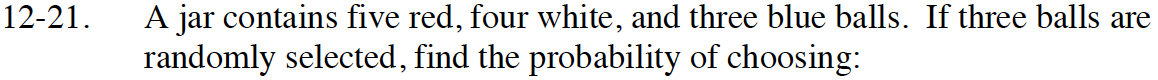Review the Math Notes box in Lesson 10.1.1 to review combinations.

There are 5 red balls, choose 2.
There are 4 white balls, choose 1.
There are 3 blue balls, choose 0.

There are 12 balls total, choose 3.

$\frac{_5C_2\;·\;_4C_1·\;_3C_0}{_{12}C_3}=\frac{2}{11}$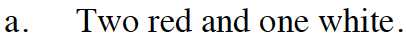See part (a).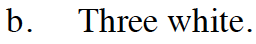See part (a).

$\frac{_5C_1\cdot _4C_1\cdot _3C_1}{_{12}C_3}=\frac{3}{11}$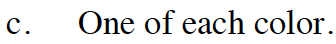Find the probabilities of all red balls,all white balls, and all blue balls.
Adding these probabilities will give you the total probability that the balls will be all the same color.

$\frac{_5C_3}{_{12}C_3}+\frac{_4C_3}{_{12}C_3}+\frac{_3C_3}{_{12}C_3}=\frac{3}{44}$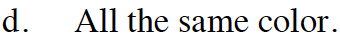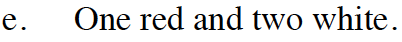See part (a).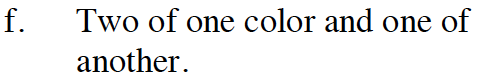Parts (c) and (d) describe the only other color combinations of balls.

$1-(\frac{3}{11}+\frac{3}{44})\ =\ \frac{29}{44}$